In Love with CodeCode Haizhou's Blog 2020-06-17T08:49:25.362Z http://www.shihaizhou.com/ Haizhou Shi Hexo Legendre Transform and Fenchel Conjugate http://www.shihaizhou.com/2020/06/17/Legendre-Transform-and-Fenchel-Conjugate/ 2020-06-17T05:57:34.000Z 2020-06-17T08:49:25.362Z Materials

# Legendre Transform

## Aim

Failed attempt. 一个非常直观的想法就是我们将 $x$ 用 $p$ 来表示，即 $x = \mathrm y ^{\prime -1}(p)$, 然后反向代回原来的函数 $\mathrm y$. 由此我们得到以下的transformed $\tilde{\mathrm y}$:

## Definition

Definition (Legendre Transformation). Legendre Transformation from a function $\mathrm y(x)$ to a new function $\mathrm y^\star (p)$ is defined as follow, where $p=\mathrm y^\prime (x)$ and no information is lost iff function $\mathrm y$ is convex (or concave, which is omitted in this blog):

## Properties

### Geometric interpretation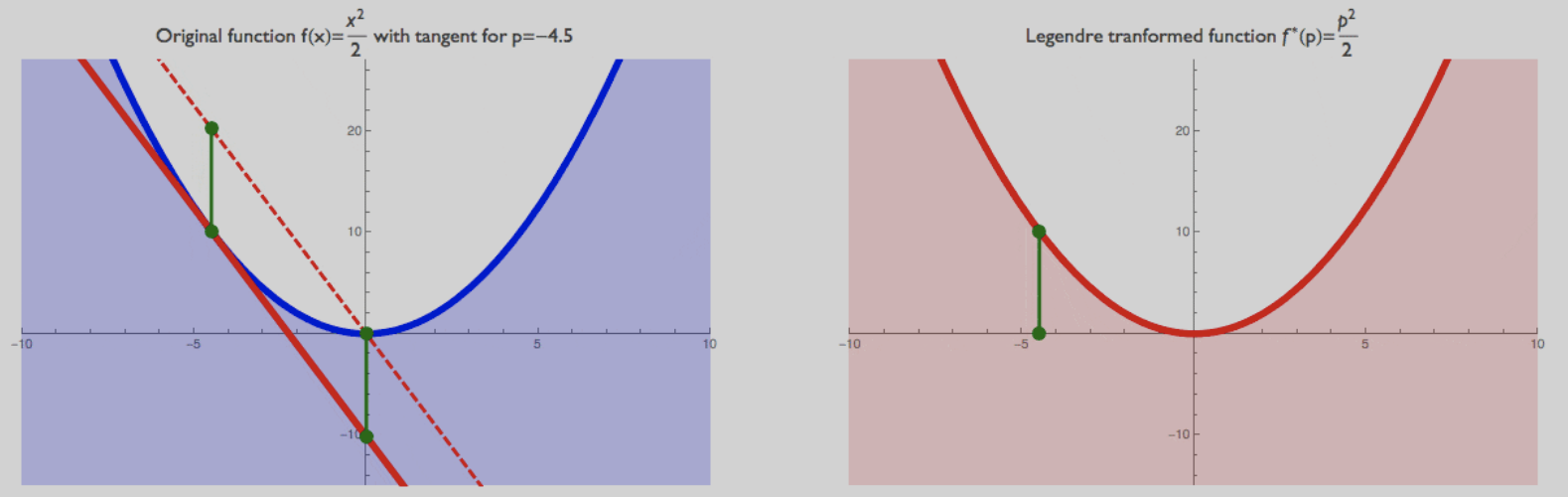### Inverse of Legendre Transformation

The Legendre Transformation of the Legendre Transformation of a function $\mathrm y$ is $\mathrm y$ itself, which is easy to prove.

# Fenchel Conjugate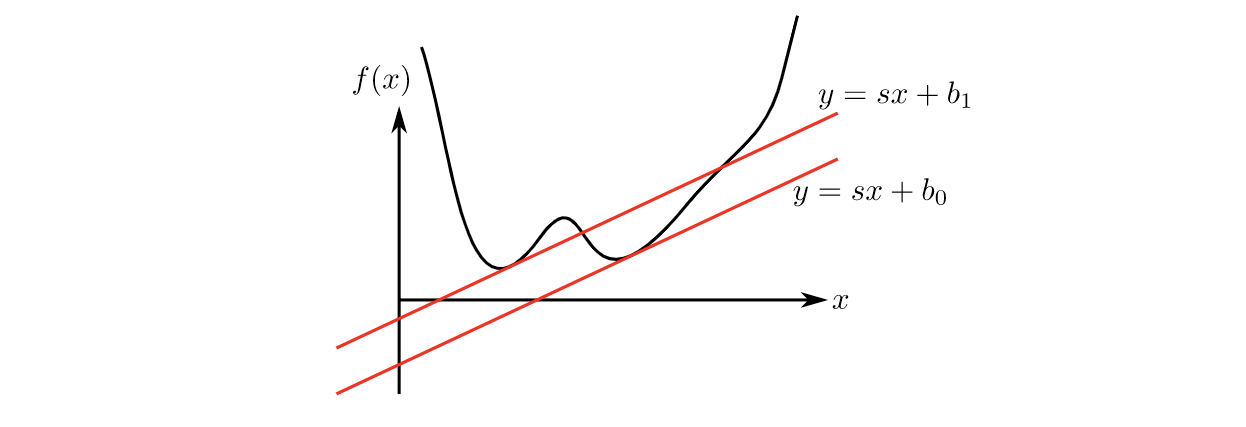This more general rule applies to non-differentiable or non-convex functions. So, when a line with slope $\mathbf s$ crosses $f(\mathbf x)$, we have:

and we want the smallest value of $b$ for all $x$. Then:

This is the Legendre-Fenchel transform, also known as convex conjugate. Note that now the transform is not reversible, i.e., you cannot get the original function by applying the transform to the transform. On the other hand, the transform of the transform is convex, even if the original function is not.

]]>
<p><strong>Materials</strong></p> <ul> <li><a href="https://www.andrew.cmu.edu/course/33-765/pdf/Legendre.pdf" target="_blank" rel="noopener

# Vanilla GAN

Deep Learning研究的问题本质上是希望学习从一个数据空间到另一个数据空间的映射；更准确地，一个distribution向另一个distribution的映射。在分类任务中，这两个分布分别为合法数据点的分布向0-1分布的映射；在回归任务中，这两个分布分别为特征空间内数据点的分布向预测空间内的分布。

GAN的核心思想在于将寻找最优generator $G$ 的过程刻画成一个由generator和discriminator两者构成的minmax game：

# f-GAN

Vanilla GAN的优化目标是JS divergence $\operatorname{JS}(P_{data} | P_G)$, 而f-GAN这篇工作则把满足一定特性的不同divergence都归在了同一个f-divergence的框架下，并且提出了对应在minmax game中优化函数的表达式。

## The f-divergence Family

A large class of different divergences are the so called f-divergences, also known as the Ali-Silvey distances. Given two distributions $P$ and $Q$ that possess, respectively, an absolutely continuous density function $p$ and $q$ with respect to a base measure $dx$ defined on the domain $\mathcal{X}$ , we define the f-divergence:

where the generator function $f: \mathbb R_+ \rightarrow \mathbb R$ is a convex, lower-semicontinuous function satisfying $f (1) = 0$.

## Estimating f-divergence

Since $f$ is convex, we have

Definition (Jensen Inequality). If $X$ is a random variable and $\varphi$ is a convex function, then following inequality holds.

where $t=T(x)$, $T: \mathcal{X} \rightarrow \mathbb{R}$. 接下来我们推导原文中略过的部分：在Legendre Transformation的语境下我们可以求出相对紧的下界 $T^*$ (注意不要和conjugate的符号混淆).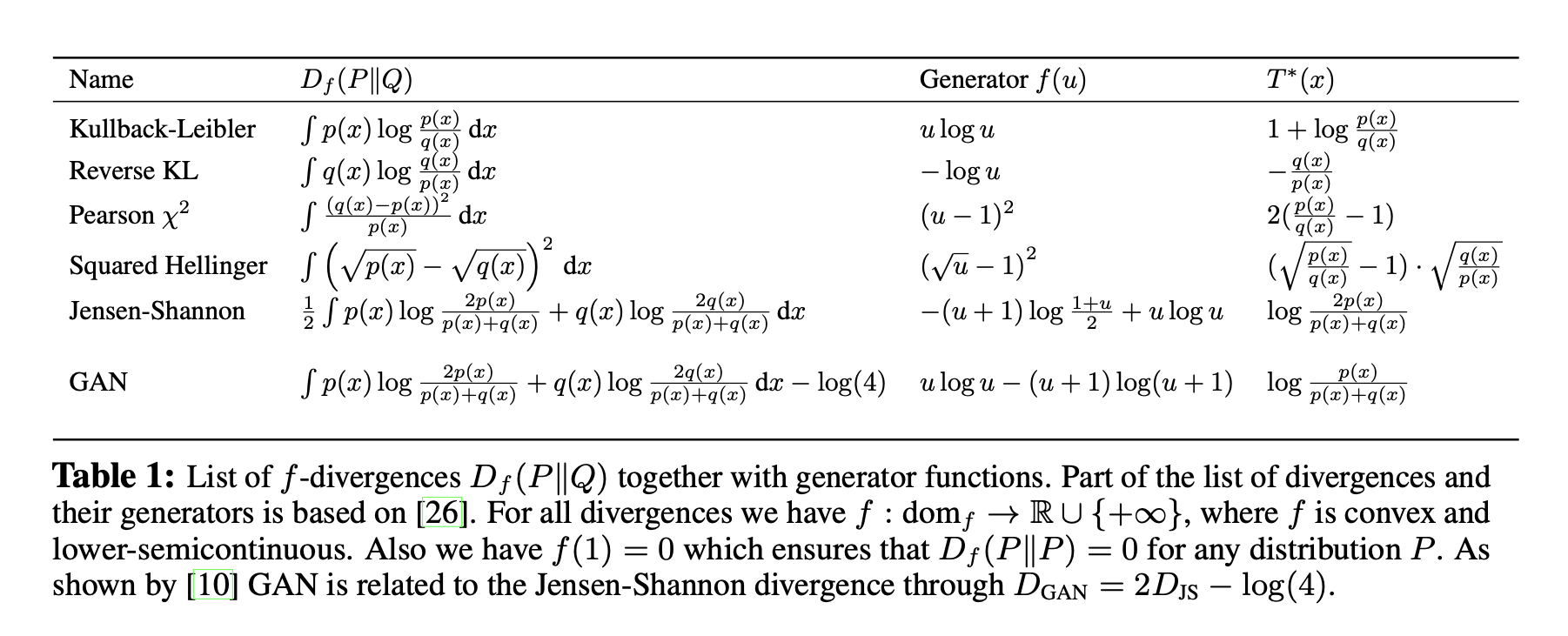## Variational Divergence Minimization (VDM)

To this end, we follow the generative-adversarial approach and use two neural networks, $Q$ and $T$ . $Q$ is our generative model, taking as input a random vector and outputting a sample of interest. We parametrize $Q$ through a vector $\theta$ and write $Q_\theta$. $T$ is our variati onal function, taking as input a sample and returning a scalar. We parametrize $T$ using a vector ω and write $T_\omega$ .

# WGAN

WGAN使用了在f-GAN family 之外的用于衡量两个分布的距离函数: Earth-Mover (EM) distance or Wasserstein-1.

Definition (Earth-Mover Distance). The Earth-Mover distance between two distribution $\mathbb P_r$ and $\mathbb P_g$ is defined as

where $\Pi (\mathbb P_r, \mathbb P_g)$ denotes the set of all joint distributions $\gamma (x,y)$ whose marginals are respectively $\mathbb P_r$ and $\mathbb P_g$. Intuitively, $\gamma (x,y)$ indicates how much “mass” must be transported from $x$ to $y$ in order to transform the distributions $\mathbb P_r$ into the distribution $\mathbb P_g$. The EM distance then is the “cost” of the optimal transport plan.

]]>
<p><strong>Materials</strong></p> <ul> <li><a href="https://math.stackexchange.com/questions/2435464/show-that-max-function-on-mathbb-rn-is-
Contrastive Learning from the Perspective of Manifold Learning http://www.shihaizhou.com/2020/06/15/Contrastive-Learning-from-the-Perspective-of-Manifold-Learning/ 2020-06-15T14:02:30.000Z 2020-06-15T14:30:15.516Z In Isomap method, the target of representation space is to keep the Geodesic Distance between arbitrary two points:

In contrastive learning, the target of representation space is that there exists a critic that can distinguish positive/negative sample pairs. The procedure of optimizing the contrastive loss is

]]>
<p>In Isomap method, the target of representation space is to keep the Geodesic Distance between arbitrary two points: </p> <script type="ma
Manifold Learning http://www.shihaizhou.com/2020/06/14/Manifold-Learning/ 2020-06-14T11:51:27.000Z 2020-06-16T11:45:38.547Z Materials

# Definition

High-dimensional datasets can be very difficult to visualize. While data in two or three dimensions can be plotted to show the inherent structure of the data, equivalent high-dimensional plots are much less intuitive. To aid visualization of the structure of a dataset, the dimension must be reduced in some way.

Manifold Learning can be thought of as an attempt to generalize linear frameworks like PCA to be sensitive to non-linear structure in data. Though supervised variants exist, the typical manifold learning problem is unsupervised: it learns the high-dimensional structure of the data from the data itself, without the use of predetermined classifications.

Definition (Geodesic Distance). A geodesic line is the shortest path between two points on a curved surface, like Earth (referring to the following figure).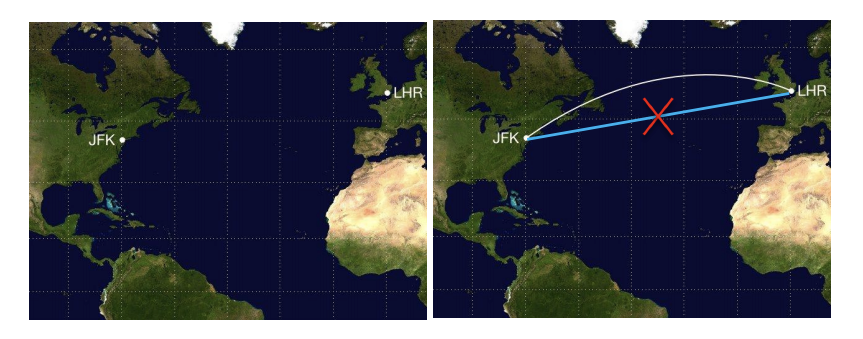# Methods

## Isomap

One of the earliest approaches to manifold learning is the Isomap algorithm, short for Isometric Mapping. Isomap seeks a lower-dimensional embedding which maintains geodesic distances between every two points.

### Estimating Geodestic Distance

We could estimate the geodestic distance by constructing an adjacency graph on which the shortest distance between two nodes is the estimation of their geodestic distance. We can set the adjacency matrix following the rule:

Isomap使用 MDS 计算映射后的坐标$y$，使得映射坐标下的欧氏距离与原来的测地线距离尽量相等.

## Locally Linear Embedding

Locally linear embedding (LLE) seeks a lower-dimensional projection of the data which preserves distances within local neighborhoods. It can be thought of as a series of local Principal Component Analyses which are globally compared to find the best non-linear embedding.

“流形在局部可以近似等价于欧氏空间”是 LLE 分析方法的出发点。LLE认为一个中心数据点 $x_i$ 能够被处于其小邻域内的点$\{x_j\}_{j \sim i}$线性重构，重构的权重可能是我们想要在表征空间上保留的geometry attributes。

# Experiments

]]>
<p><strong>Materials</strong></p> <ul> <li><a href="https://scikit-learn.org/stable/modules/manifold.html" target="_blank" rel="noopener">sk
Understanding Contrastive Representation Learning http://www.shihaizhou.com/2020/06/11/Understanding-Contrastive-Representation-Learning-through-Alignment-and-Uniformity-on-the-Hypersphere/ 2020-06-11T13:14:56.000Z 2020-06-15T11:09:08.003Z QA

Q1. For classification tasks, when we use contrastive learning as the self-supervised objective, the uniformity of the representations is optimized but how the linear-separability is naturally optimized along with uniformity?
A1. In my opinion, the reason that class concentration happens is ultimately the inductive bias of neural networks. Afterall, unrestricted function class, there definitely are encoders that are very aligned and uniform, but gives useless features, in terms of linear classification at least. If you believe the inductive bias of NN tends to lead to “smooth” solutions, then intuitively class concentration happens. It is certainly difficult to argue about this formally though.

Q2. For tasks requiring structured output (e.g. reconstruction), I could understand that uniformity is desired since we want the representation to be as different as possible so that when constructing output, different samples are not going to be confused. Then I wonder if the contrastive method is better than the Autoencoder-based method (in this scenario). My assumption is “no” since the contrastive method also pushes similar samples away from each other, while it’s against our instinct that similar inputs shall have similar representations.
A2. I’d say it depends on how you choose positive pairs in contrastive learning. Surely this could be true if you ask two random crops of the same image to have the same features.

]]>
Disentangled Representation Learning via Mutual Information Estimation http://www.shihaizhou.com/2020/06/10/Learning-Disentangled-Representations-via-Mutual-Information-Estimation/ 2020-06-10T09:03:39.000Z 2020-06-10T10:39:59.943Z Materials

# Method Description

Given a pair of images sharing some attributes, we aim to create a low-dimensional representation which is split into two parts: a shared representation that captures the common information between the images and an exclusive representation that contains the specific information of each image.

Two stages of training. First, the shared representation is learned via cross mutual information estimation and maximization. Secondly, mutual information maximization is performed to learn the exclusive representation while minimizing the mutual information between the shared and exclusive representations.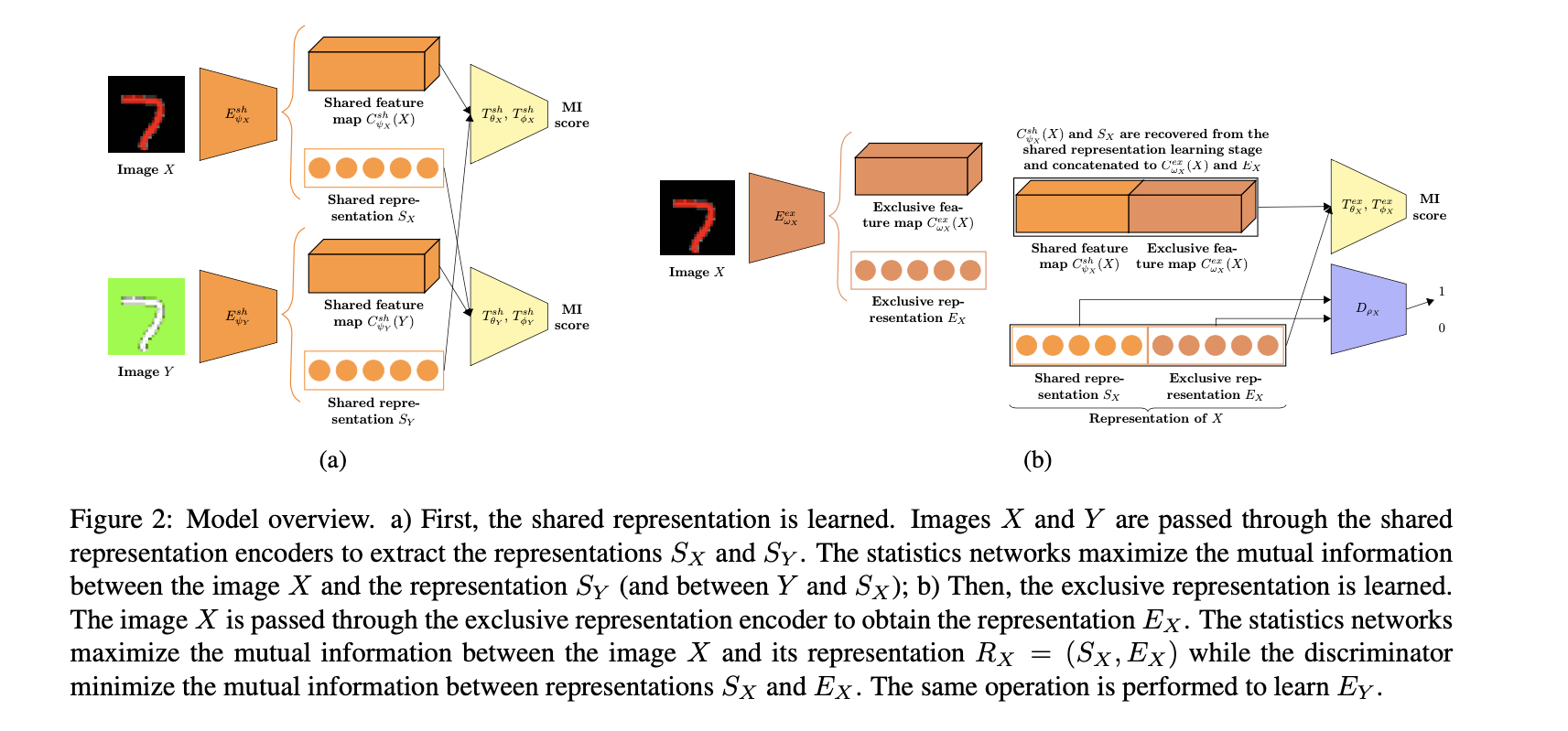# Experiments

## Question

Dear Eduardo Hugo Sanchez,

After reading your wonderful paper “Learning Disentangled Representations via Mutual Information Estimation”, I have one question regarding the setup of your training procedure, which is:

How do you decide between which two images the MI is maximized during training? Say in Colorful MNIST, if you put the images of the same digit together and follow your obejective, then can I conclude that you’re actually telling the model to learn a (linear, in some cases) seperable representation regarding the digit classification? Below is how I come to this conclusion:
If we are maximizing the MI between all the images containing the same digit, then during the process of MI maximization, we will shuffle the whole batch of the data in order to form the negative samples, which will be feed into the critic function. Since the critic function has to distinguish the samples like (X,Y)=(black7, red7) and the shuffled samples like (X,Y’)=(black7, yellow10), we are explicitly telling the model to classify the digits.
If we train the whole network in a totally unsupervised way, i.e., training sample pairs like (X,Y)=(black7, yellow10) are randomly showing up asking the model to learn the shared information between them, how the method is able to learn the disentangled representations is really confusing me…

Furthermore, did you do the ablation study of the “cross mutual information maximization” technique? How did it go?

]]>
<p><strong>Materials</strong></p> <ul> <li><a href="https://arxiv.org/abs/1912.03915" target="_blank" rel="noopener">Learning Disentangled R
InfoAE - Unpublished http://www.shihaizhou.com/2020/06/09/InfoAE-Unpublished/ 2020-06-09T04:17:07.000Z 2020-06-09T08:21:02.053Z Materials

# InfoAE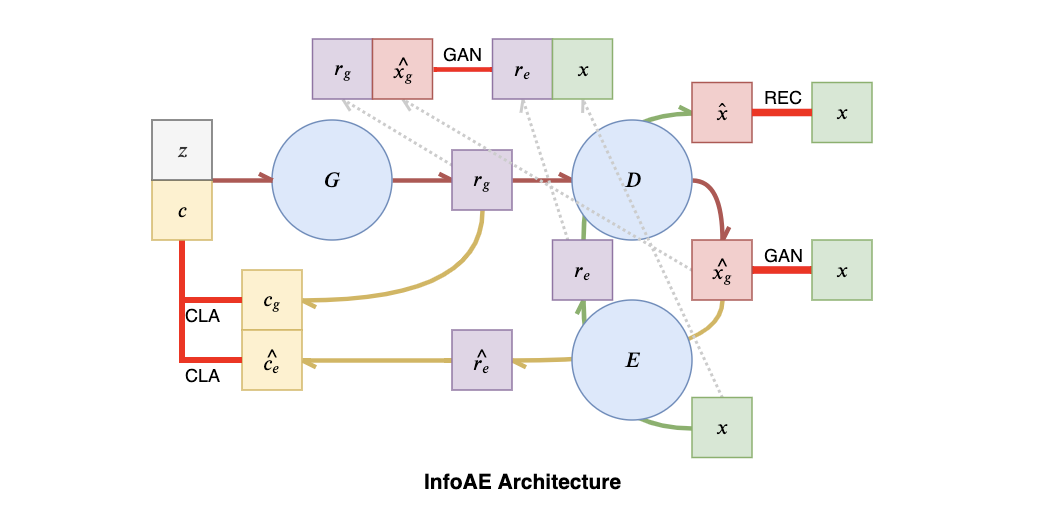InfoAE可以分为Conditional GAN和Autoencoder两个部分，如上图所示。

Conditional GAN的部分对应于上图的红色流程+黄色流程：首先 $z$ 是prior random noise，$c$ 是latent code；在实验中取 $c$ 为 $K=10$ 的one-hot coding. 经过Generator Network $G$ 之后映射到了latent representation space $r$；接着 $r$ 通过Decoder Network得到了fake sample $\hat {x_g}$. 将 fake sample 和 true sample进行对比从而得到了GAN loss. 同时因为该方法是为了下游的分类任务服务，所以我们希望Encoder能够将分类信息进行编码。但是对于一个完全无标签的非监督学习样本$x$来说，我们是没有办法显式地为他进行分类的，唯一编码了分类信息的变量就是latent code $c$. 因此我们将得到的中间表示 $r$ 重新经过一个Classifier Network，将得到的分类结果重新映射回 $c$.

Autoencoder部分就是最简单的Reconstruction Error，对应于上图中的绿色流程。

# Experiments

We have evaluated the model on MNIST dataset and received outstanding results. InfoAE is trained on MNIST training data without any labels. After trainning, We encoded the test data with Encoder, E and got classification label with the Classifier, C. Then we clustered the test data according to label and received classification accuracy of 98.9 (±.05), which is better than the popular methods as shown in Table 1.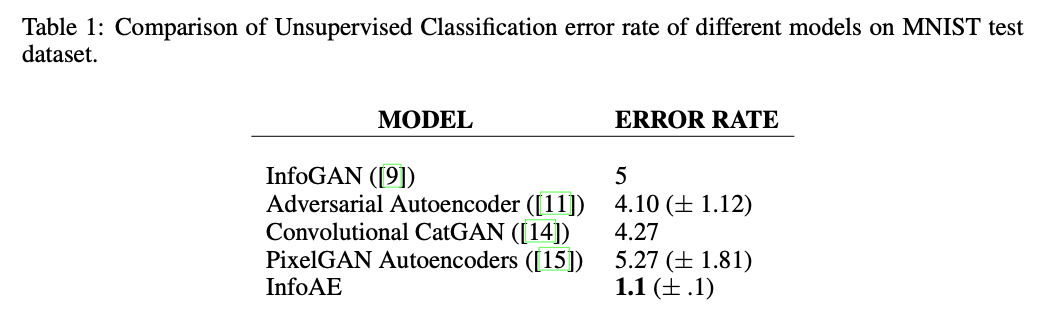]]>
<p><strong>Materials</strong></p> <ul> <li><a href="https://arxiv.org/abs/1904.08613" target="_blank" rel="noopener">arxiv preprint paper “D
InfoGAN http://www.shihaizhou.com/2020/06/08/InfoGAN/ 2020-06-08T11:37:22.000Z 2020-06-09T04:32:47.958Z Materials

# Motivation

Problem of unsupervised representation learning. While unsupervised learning is ill-posed because the relevant downstream tasks are unknown at training time, a disentangled representation, one which explicitly represents the salient attributes of a data instance, should be helpful for the relevant but unknown tasks. Thus, to be useful, an unsupervised learning algorithm must in effect correctly guess the likely set of downstream classification tasks without being directly exposed to them.

How to learn disentangled representation in this paper. In this paper, we present a simple modification to the generative adversarial network objective that encourages it to learn interpretable and meaningful representations. We do so by maximizing the mutual information between a fixed small subset of the GAN’s noise variables and the observations, which turns out to be relatively straightforward.

# Methods

## Mutual Information for Inducing Latent Codes

In this paper, rather than using a single unstructured noise vector, we propose to decompose the input noise vector into two parts: (i) $z$, which is treated as source of incompressible noise; (ii) $c$, which we will call the latent code and will target the salient structured semantic features of the data distribution.

We provide the generator network with both the incompressible noise z and the latent code c, so the form of the generator becomes $G(z, c)$. However, in standard GAN, the generator is free to ignore the additional latent code $c$ by finding a solution satisfying $P_G(x|c) = P_G(x)$. 为了解决trivial codes的问题，我们认为code $c$ 和生成结果generator distribution $G(z, c)$之间有较强的dependency，也就是说 $I(c; G(z,c))​$ 应当较高. 最终，InfoGAN优化的目标为以下：

## Variational Mutual Information Maximization

PASS. We will use InfoMax method to reproduce the experimental results.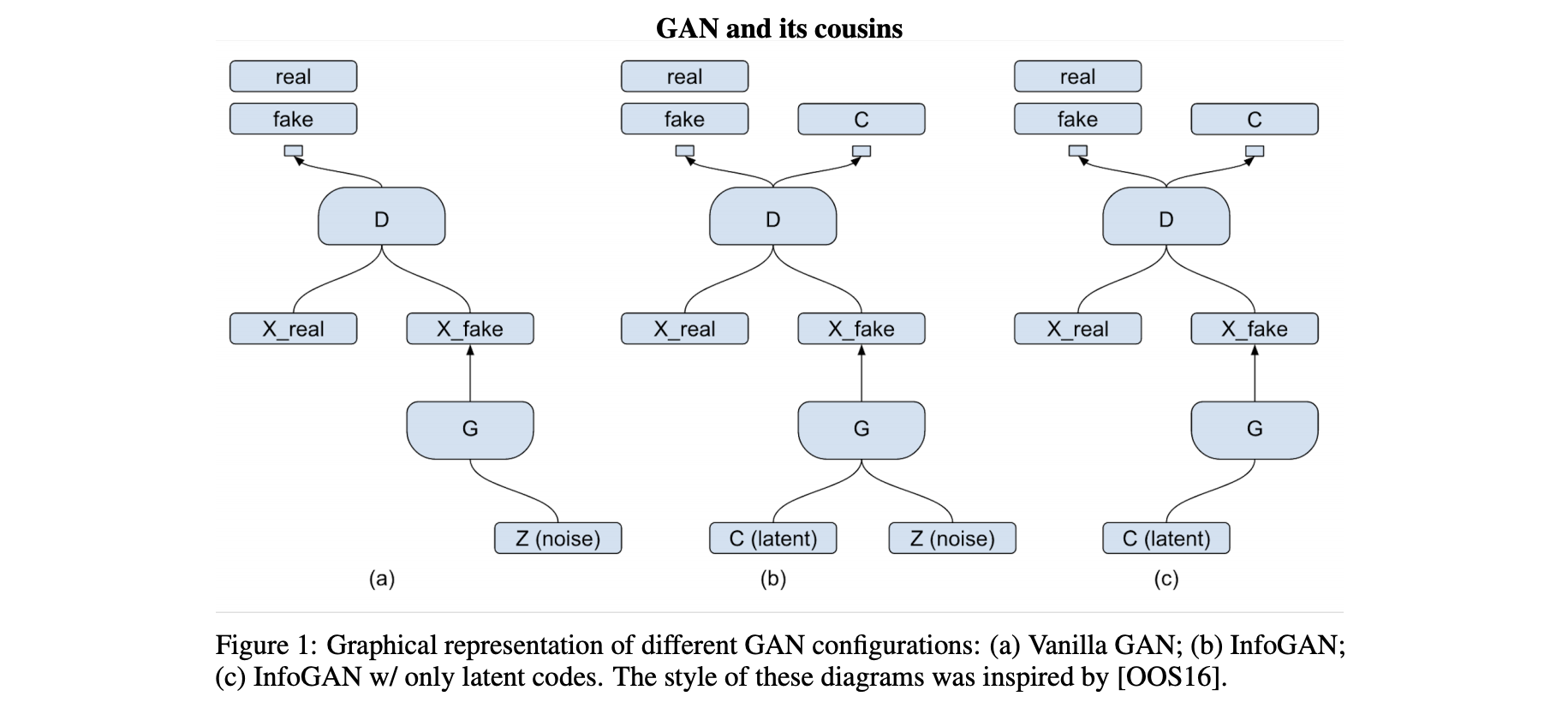# Experiments

GAN的实验部分通常都比较偏经验性，并没有太好的Numerical Metrics来进行比较。值得一提的是，InfoGAN和之前看到的一篇未投稿工作一样，在MNIST数据集上验证时将latent code设置成了$K=10$ 的one-hot coding。我认为在这种setting下，representation进入下游分类任务取得很好的成绩是因为10-way classification是关于下游任务非常重要的信息泄露。以下我们记录一下使用InfoMax来优化InfoGAN的实验。

]]>
<p><strong>Materials</strong></p> <ul> <li><a href="https://arxiv.org/abs/1606.03657" target="_blank" rel="noopener">InfoGAN paper</a></li>
Matplotlib Cookbook http://www.shihaizhou.com/2020/06/07/Matplotlib-Cookbook/ 2020-06-07T08:09:35.000Z 2020-06-07T15:17:27.947Z 3D Drawing

## Dynamic Drawing

In Jupyter Notebook: use display in Ipython

]]>
Discriminative Clustering by Regularized Information Maximization http://www.shihaizhou.com/2020/06/04/Discriminative-Clustering-by-Regularized-Information-Maximization/ 2020-06-04T11:02:37.000Z 2020-06-07T15:13:29.158Z Materials

# Motivation

It is folklore knowledge that maximizing MI does not necessarily lead to useful representations. Already Linsker (1988) talks in his seminal work about constraints, while a manifestation of the problem in clustering approaches using MI criteria has been brought up by Bridle et al. (1992) and subsequently addressed using regularization by Krause et al. (2010).

We propose a principled probabilistic approach to discriminative clustering, by formalizing the problem as unsupervised learning of a conditional probabilistic model.

We identify two fundamental, competing quantities, class balance and class separation, and develop an information theoretic objective function which trades off these quantities.

Our approach corresponds to maximizing mutual information between the empirical distribution on the inputs and the induced label distribution, regularized by a complexity penalty. Thus, we call our approach Regularized Information Maximization (RIM).

# Experimental Results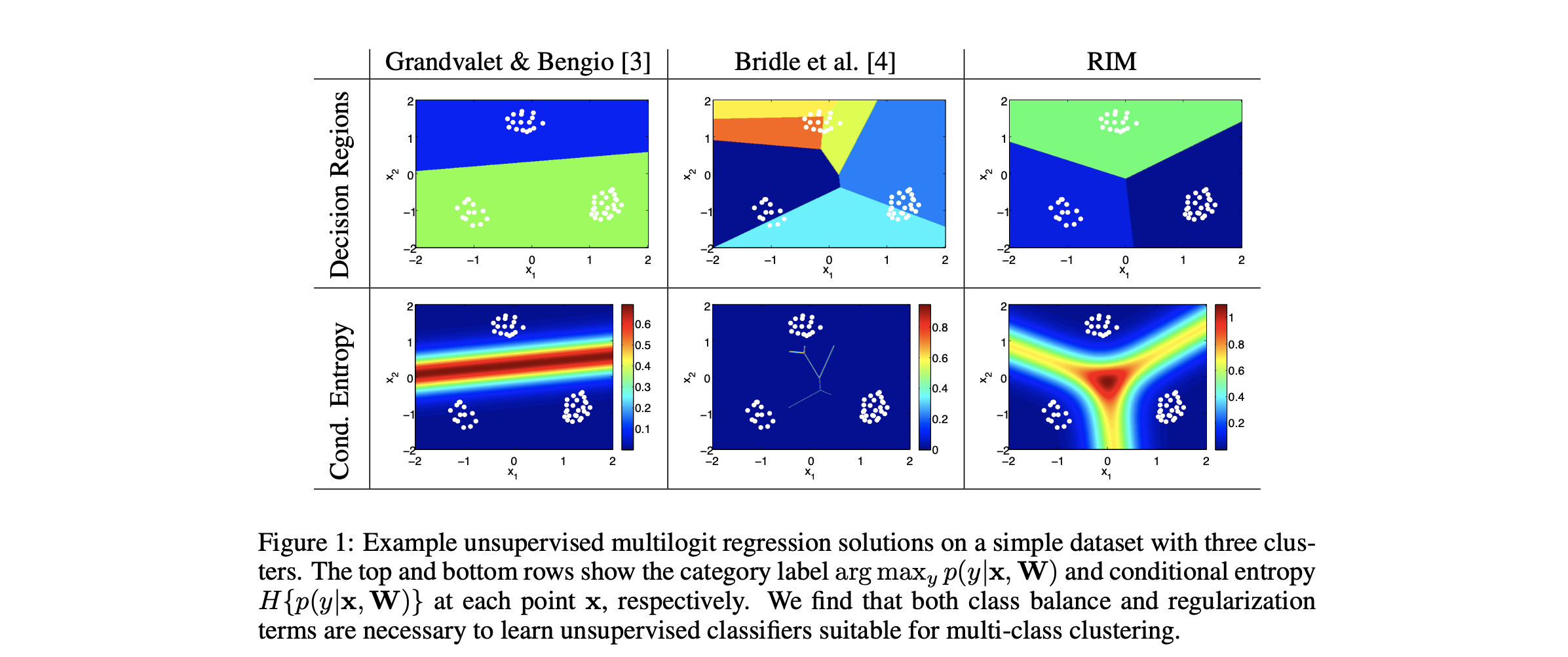]]>
<p><strong>Materials</strong></p> <ul> <li><a href="https://papers.nips.cc/paper/4154-discriminative-clustering-by-regularized-information-m
Deep Graph InfoMax http://www.shihaizhou.com/2020/06/02/Deep-Graph-InfoMax/ 2020-06-02T09:07:44.000Z 2020-06-02T09:08:44.029Z Motivation

Deep Graph Infomax (DGI) is a general approach for learning node representations within graph-structured data in an unsupervised manner. In contrast to most prior approaches to unsupervised learning with GCNs, DGI does not rely on random walk objectives, and is readily applicable to both transductive and inductive learning setups.

## Structure of Introduction

• Generalizing node-level representation learning is important, large-scale graph data is often with no labels. -> thus unsupervised representation learning method is much more important.
• Existing methods most rely on random walk-based objective, which
• over-emphasize proximity information at the expense of structural information
• is highly influenced by hyperparameter choice
• when introduced with stronger encoder, hard to tell whether the representation has meaningful signals.
• Deep InfoMax on image data, maximize the global/local mutual information. This encourages the encoder to carry the type of information that is present in all locations (and thus are globally relevant), such as would be the case of a class label.
• We are the first work applying it to the graph data.
]]>
Data Simulation Cookbook http://www.shihaizhou.com/2020/05/31/Data-Simulation/ 2020-05-31T11:47:14.000Z 2020-06-07T08:32:29.399Z Multi-Variate Gaussion

Need to provide the mean and covariance matrix.

## 2D-ring

Produce a ring for given $(x, y, r)$ triplet. The first one use the famous formula adopted in VAE.

## 3D-globule

Produce len(centers) gaussian globules.

]]>
Pytorch - CNNs http://www.shihaizhou.com/2020/05/28/Pytorch-CNNs/ 2020-05-28T13:39:09.000Z 2020-06-10T15:51:56.349Z Materials

# Functions

### torch.nn.Conv2d

Pytorch和tensorflow中（也和我们一般理解的）的图像表示形式不同：torch中的channel维在height和width之前，所以input的tensor形状为$(N,C_{\text{in}}, H, W)$.

]]>
<p><strong>Materials</strong></p> <ul> <li><a href="https://pytorch.org/tutorials/beginner/blitz/cifar10_tutorial.html" target="_blank" rel=
Contrastive Predictive Coding http://www.shihaizhou.com/2020/05/28/Contrastive-Predictive-Coding/ 2020-05-28T02:17:22.000Z 2020-06-08T15:44:27.967Z Materials

• paper “Contrastive Predictive Coding”

The key insight of our model is to learn such representations by predicting the future in latent space by using powerful autoregressive models. We use a probabilistic contrastive loss which induces the latent space to capture information that is maximally useful to predict future samples.

Predictive coding在信号处理领域是常见的unsupervised learning方法. 本文的方法流程为：

• First, we compress high-dimensional data into a much more compact latent embedding space in which conditional predictions are easier to model.
• Secondly, we use powerful autoregressive models in this latent space to make predictions many steps in the future.
• Finally, we rely on Noise-Contrastive Estimation for the loss function.
]]>
<p><strong>Materials</strong></p> <ul> <li>paper “Contrastive Predictive Coding”</li> </ul> <p>The key insight of our model is to learn such
Information Competing Process for Learning Diversified Representations http://www.shihaizhou.com/2020/05/27/Information-Competing-Process-for-Learning-Diversified-Representations/ 2020-05-27T06:57:20.000Z 2020-05-28T02:17:06.212Z Materials

# Motivation

Aiming to enrich the information carried by feature representations, ICP separates a representation into two parts with different mutual information constraints. The separated parts are forced to accomplish the downstream task independently in a competitive environment which prevents the two parts from learning what each other learned for the downstream task. Such competing parts are then combined synergistically to complete the task.

## Representation Collaboration

The Competitive Collaboration method is the most relevant to our work. It defines a three-player game with two competitors and a moderator, where the moderator takes the role of a critic and the two competitors collaborate to train the moderator. Unlike Competitive Collaboration, the proposed ICP enforces two (or more) representation parts to be complementary through different mutual information constraints for the same downstream task by a competitive environment, which endows the capability of learning more discriminative and disentangled representations.

# Methods

## Seperating Representations

Directly separate the representation $r$ into two parts $[z, y]$. Specifically, we constrain the information capacity of representation part $z$ while increasing the information capacity of representation part $y$.

## Representation Competition

ICP prevents $z$ and $y$ from knowing what each other learned for the downstream task, which is realized by enforcing $z$ and $y$ independent of each other.

where $\alpha^\prime > 1$ and $\beta^\prime < 1$. 如果把$z$和$y$分别看作数据的两个view，那么中间两项就代表不同view和$X$ 之间的相关度；第一项代表全局representation和input之间的相关度；而最后一项就是view之间的InfoMin项。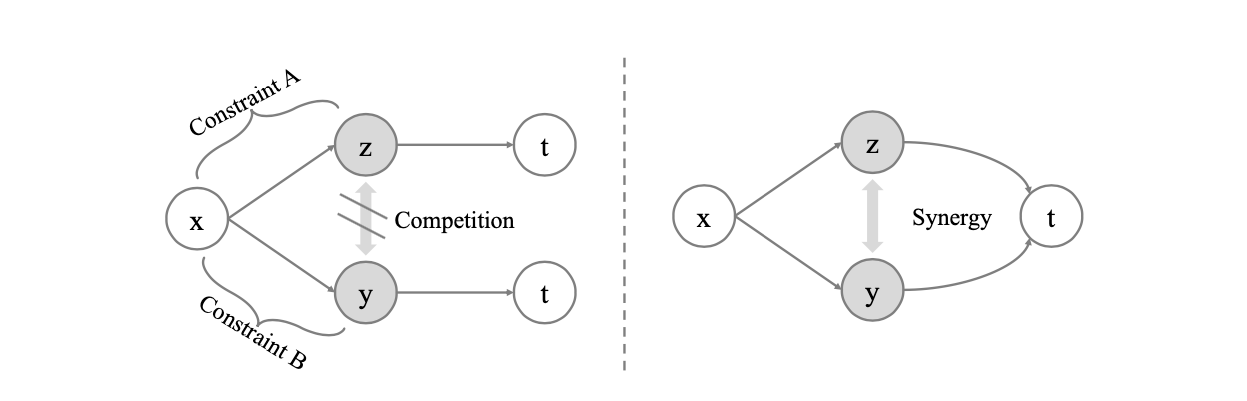## Minimizing MI

Let $Q(z)$ be a variational approximation of $P(z)$, we have:

which enforces the extracted $z$ conditioned on $x$ to a predefined distribution $Q(z)$ such as a standard Gaussian distribution.

]]>
<p><strong>Materials</strong></p> <ul> <li><a href="https://arxiv.org/abs/1906.01288" target="_blank" rel="noopener">NIPs-2019 paper “Inform
InfoBal - Ideas (failed) http://www.shihaizhou.com/2020/05/26/InfoBal-Ideas/ 2020-05-26T14:25:21.000Z 2020-06-10T10:43:43.561Z Materials

# Motivation

CMC的动机在于：重要的信息在多个view中被共享，所以我们应当最大化多个view的mutual information. 在CMC作者的延续性工作中，他认为只考虑view-invariant的信息是不够的，最佳的信息应当是和下游任务高度相关的，因此提出了InfoMin principle, 其核心思想是在input的两个view连同整体input和下游任务标签的MI保持一致的前提下，尽量使两个view之间的MI降低。而ICLR-2020的文章则将以往提出的三个MI优化目标（分别为global/local MI, CMC, CPC）都归入了统一的multiview理论框架下，认为这三个优化目标是这个框架下平行的优化目标。

# Theory

## Problem Description

Suppose the input dataset is $X$. Consider the simplest setting of only 2 views $V_1$ and $V_2$ of $X$, which is parameterized by $\theta_1$ and $\theta_2$:

In original InfoMax principle, the objective of learning representation of single view is to maximize:

When there are multiple views involved, we want them to be simultaneously maximized:

In InfoMin principle, the objective is to minimize the MI between different views under certain (complicated) constraints.

The above two objectives without constraints may not be so favorable analytically, since they both have trivial solutions which result in useless representation.

• For the original InfoMax principle: let function $g$ be bijective function, all the information of $X$ is preserved and the MI is maximized, no good form of representation is learned.
• For the multiview InfoMin principle: let $V_1$ and $V_2$ output constant vectors, and their MI is 0, which is minimized.

## InfoBal Objective

To solve this problem, notice that there exists an inequality:

which yields:

So we can change our objective of representation learning with $M$ views to maximizing the following:

where $\Theta=\{\theta_1, \theta_2, \cdots, \theta_M\}$ represents the set of the view encoders, and is guaranteed bounded.

The reason why this formula is better than previous ones is that it helps the model to balance the information capacity and multiview diversity of the representation. And also in the two extreme cases where two single objectives can’t deal with morbid representation, the new objective function equally hates them:

In the first morbid scenario, when every $V_i$ preserves all the info about $X$, i.e.,

which is quite favorable, since the views (representations) are super powerful. While without constraints, we can assume the happening of the worst: each view is exactly identical, which makes the multiview setting not learning diverse representations. This case is discouraged by our new objective:

In the second morbid scenario, when MI across the views are minimized, which means $V_i$ is irrelevant to each other, i.e., $I(V_i, V_j)=0$, this InfoMin situation could lead to extreme loss of information:

while in our objective, this is also discouraged:

## Global/Local InfoBal

The final representation $R$ is the result of view aggregation:

In global/local MI maximization, the target is to maximize the Mutual Information between the global and the local representation of the data:

which lacks theoretical derivation why this is a more favorable objective. We could also do this adaptation to our new objective by replacing original input $X$ with final representation $R$:## Weighted InfoBal

Another potential optimiztion for InfoBal is to assign two weight matrix: view weight and correlation weight (这个名字不确定).

## Optimizing InfoBal

One way is to adversarially optimize the InfoBal training objective, since the InfoMin is a minmax problem.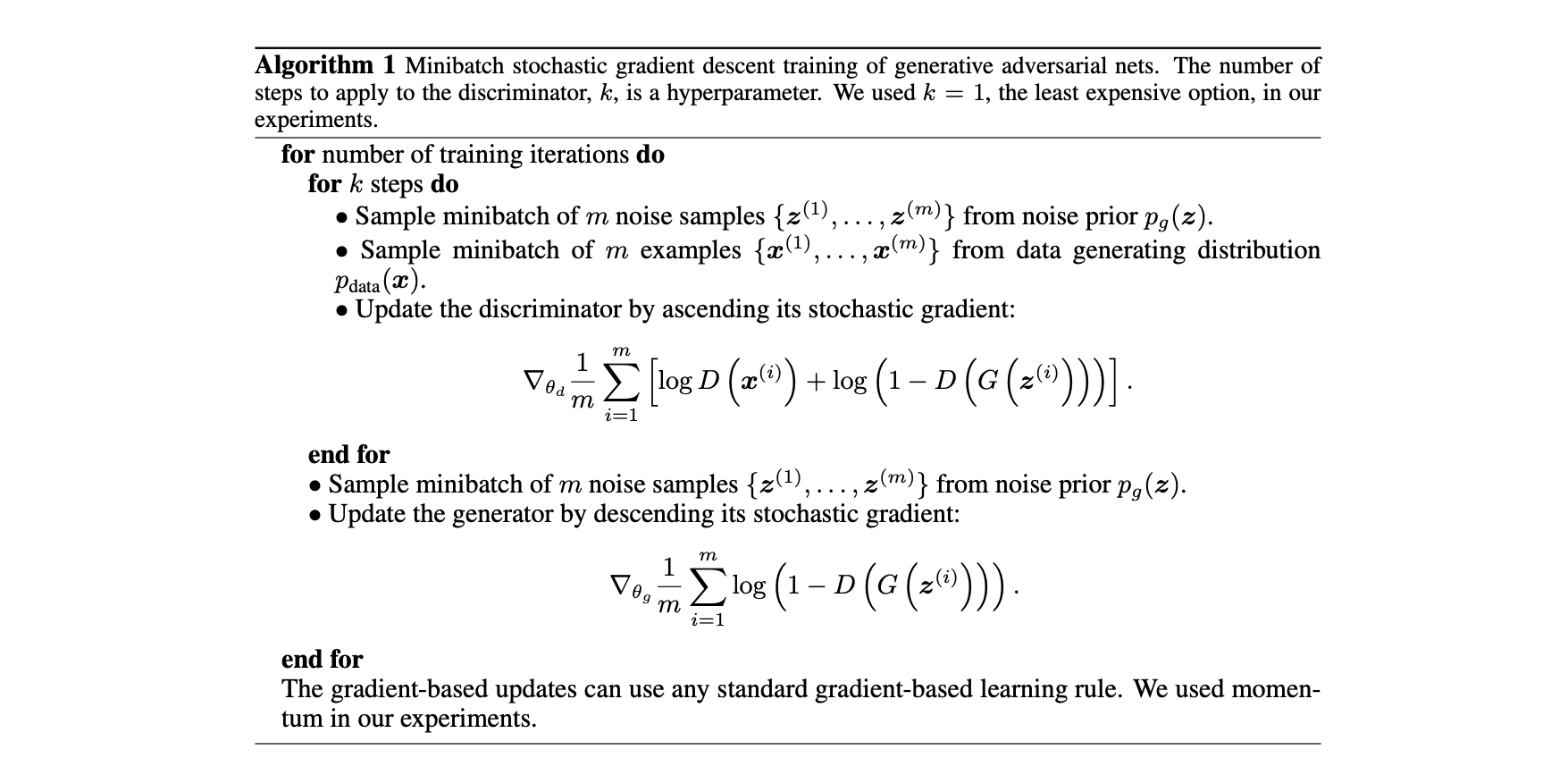Another way is to found the upper bound of the objective and minimize the upper bound, which is introduced in another similar paper (refer to NIPs-2019 paper “Information Competing Process for Learning Diversified Representations”). This one involves the knowledge of variational inference, which will be investigated in the future.

# Experiments

• View Encoder: 从raw input进行多个view的编码模块
• View Aggregator: 从view representation聚合成最终representation的模块
• InfoMax critic: 用于MI maximization的parameterized DV representation模块
• InfoMin discriminator: 最小化view之间的MI的discriminator模块We conducted the experiments on CIFAR10 dataset using the 2-view setting: instead of simultaneously maximizing the MI between the representation and the feature map vectors, we split the feature map into top/bottom parts and maximize the MI.

To evaluate the quality of the final representation, we plug the representation into a one-layer neural classifier as DIM does. We train the classifier for 4 epochs, which is by test converged, and evaluate the accuracy of the classifier.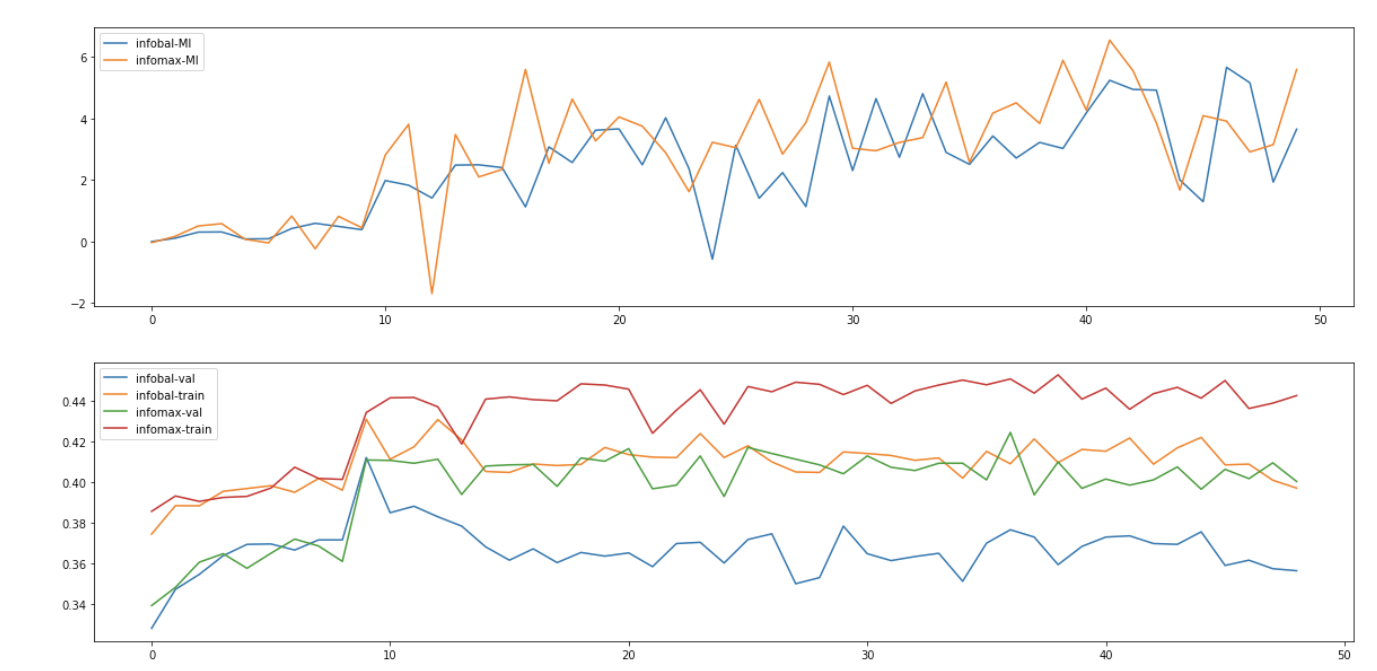Change into JSD critic function, and we have the result: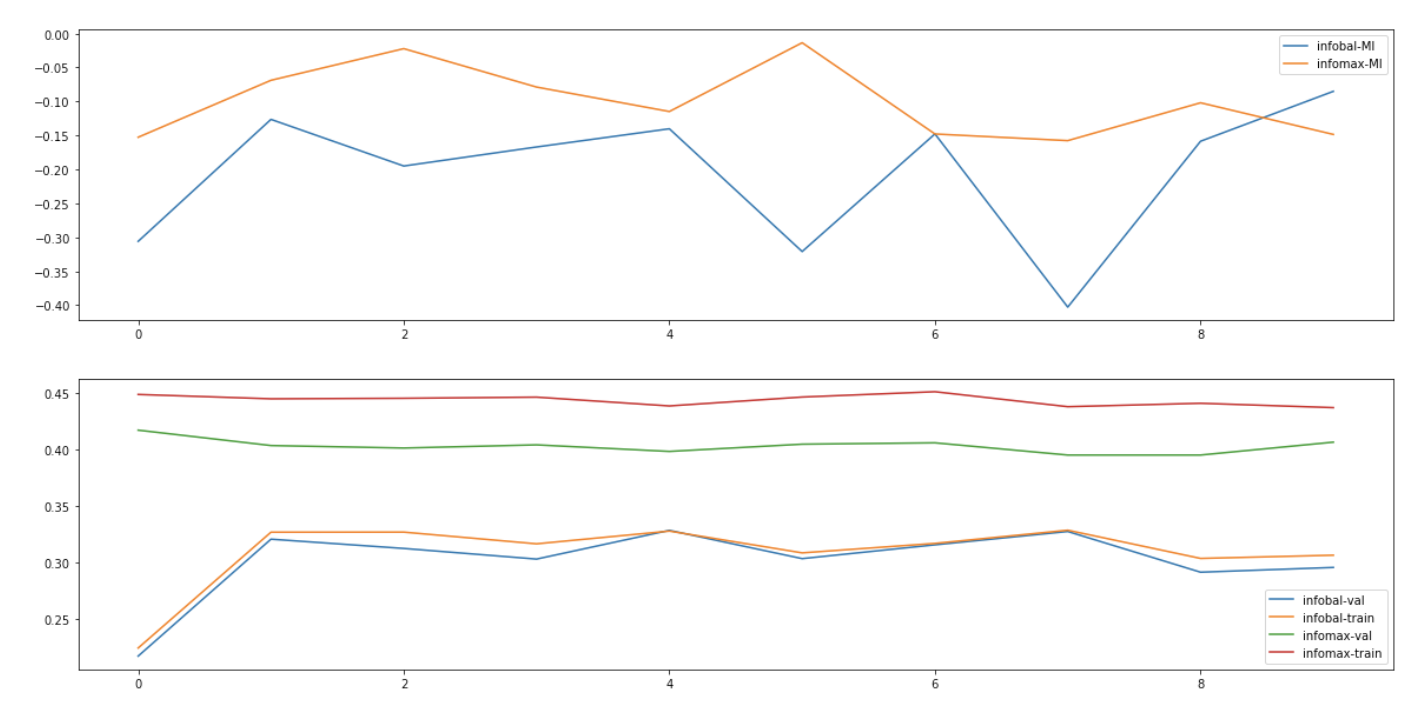When trained in much longer period, we can even find out that the InfoBal objective is constantly doing harm to the representation quality.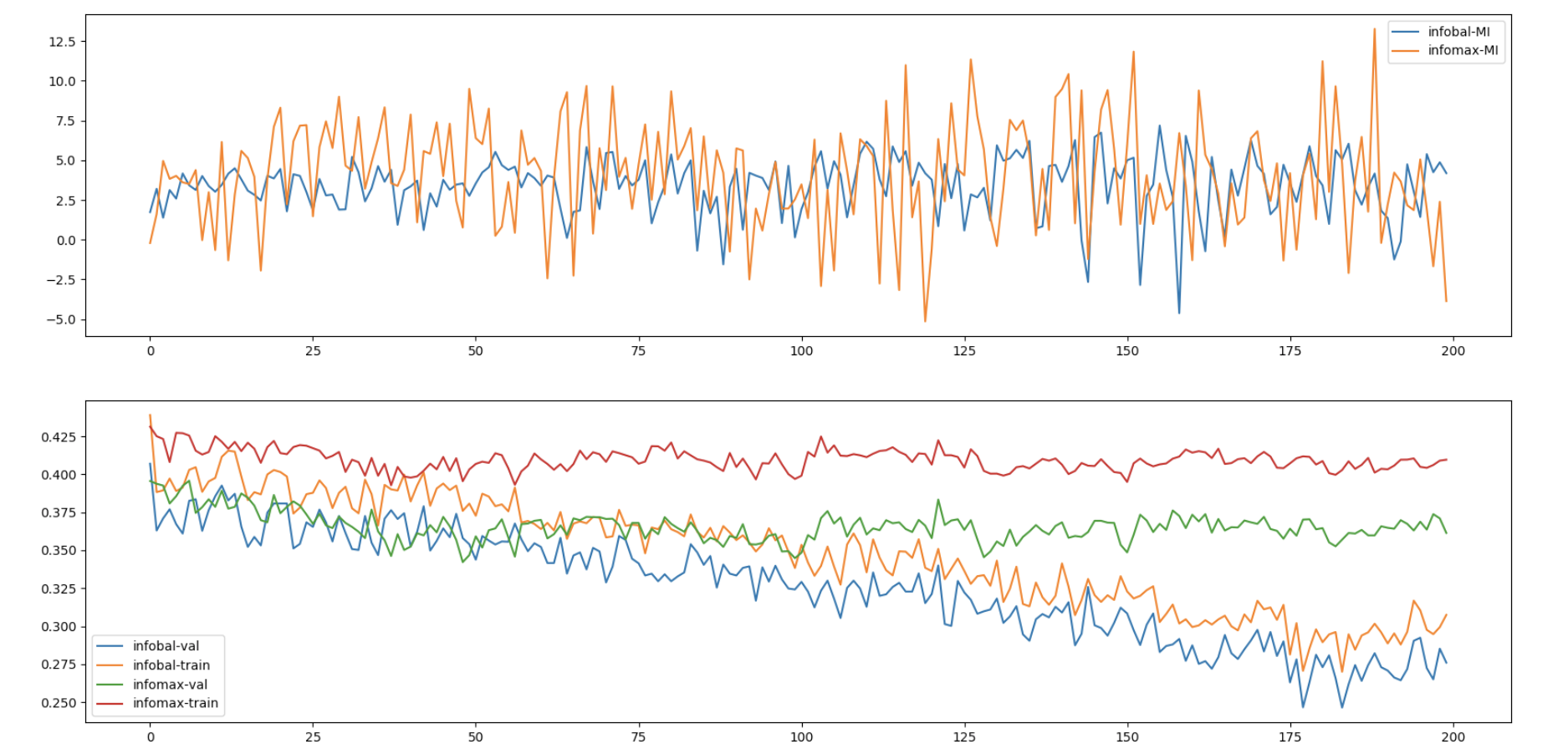The figure above is the experimental result, about which we have some questions and concerns:

• The lower bound of MI is increasing along training, while the downstream task accuracy is not improved (as much) as the MI estimate.
• The improvement compared to the original starting points is rather marginal.
• InfoBal objective is constantly hurting the performance of the representation learning model, despite the fact that the lower bound MI estimate value is nearly the same, which also implies that the MI is not so relevant to the representation quality.

Up to now, we can conclude that the new objectice InfoBal is a failed attempt. One thing worth trying though, is to reimplement the DIM’s global/local objective in multiple (way more than 2) views and shed some light on our future exploration.

]]>
<p><strong>Materials</strong></p> <ul> <li><a href="https://arxiv.org/abs/1907.13625" target="_blank" rel="noopener">ICLR-2020 paper “On Mut
What Makes for Good Views for Contrastive Learning http://www.shihaizhou.com/2020/05/26/What-Makes-for-Good-Views-for-Contrastive-Learning/ 2020-05-26T02:17:14.000Z 2020-05-28T07:16:30.680Z Materials

# Motivation

Despite the success of the Contrastive Multiview Coding (CMC), the influence of different view choices has been less studied. In this paper, we use empirical analysis to better understand the importance of view selection, and argue that we should reduce the mutual information (MI) between views while keeping task-relevant information intact. To verify this hypothesis, we devise unsupervised and semi-supervised frameworks that learn effective views by aiming to reduce their MI.

## Structure of Introduction

• CMC relies on the fundamental assumption that important information is share across views, which means it’s view-invariant.
• Then which viewing conditions should it be invariant to?
• We therefore seek representations with enough invariance to be robust to inconsequential variations but not so much as to discard information required by downstream tasks.
• We investigate this question in two ways.
• Optimal choice of views depends critically on the downstream task.
• For many common ways of generating views, there is a sweet spot in terms of downstream performance where the mutual information (MI) between views is neither too high nor too low.
• InfoMin principle: A good set of views are those that share the minimal information necessary to perform well at the downstream task.

# Methods

Definition 4.1. (Sufficient Encoder) The encoder $f_1$ of $v_1$ is sufficient in the contrastive learning framework if and only if $I(v_1; v_2) = I(f_1(v_1); v_2)$.

Definition 4.2. (Minimal Sufficient Encoder) A sufficient encoder $f_1$ of $v_1$ is minimal if and only if $I(f_1(v_1);v_1) \leq I(f(v_1);v_1) \forall f$, that are sufficient. Among those encoders which are sufficient, the minimal ones only extract relevant information of the contrastive task and will throw away other information.

Definition 4.3. (Optimal Representation of a Task) For a task $\mathcal T$ whose goal is to predict a semantic label $y$ from the input data $x$, the optimal representation $z^\star$ encoded from $x$ is the minimal sufficient statistic with respect to $y$. 以上说明了$z^\star$保留了所有用于和task $\mathcal T$相关的信息，因此被称作optimal的。

## InfoMin Principle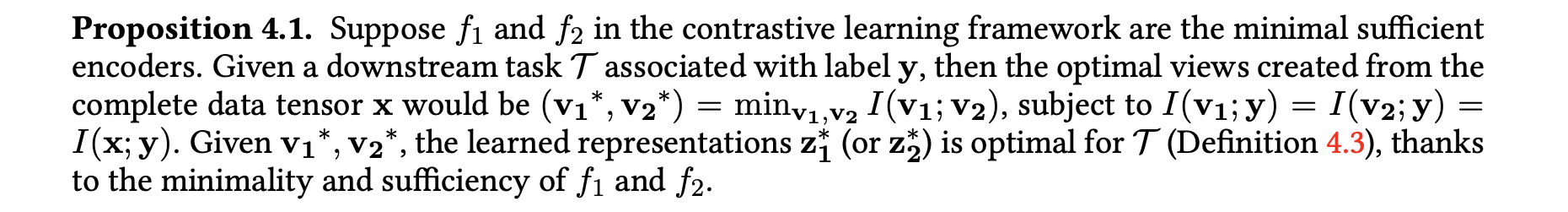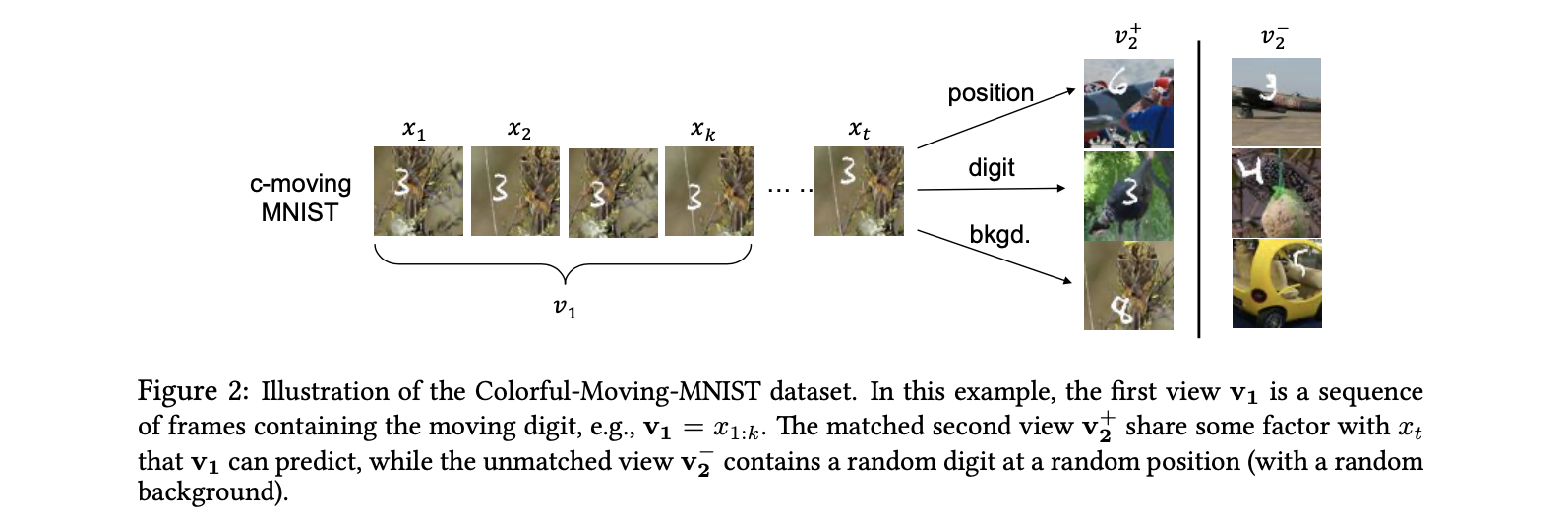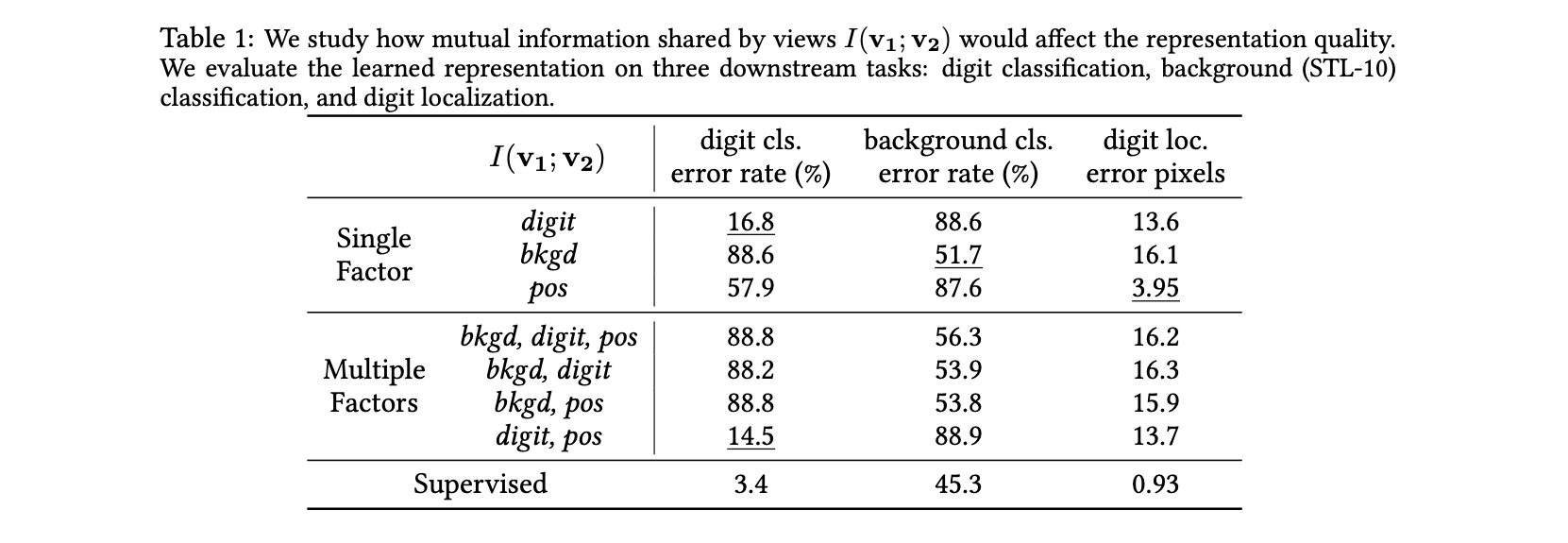## Unsupervised InfoMin]]>
<p><strong>Materials</strong></p> <ul> <li><a href="https://arxiv.org/abs/2005.10243" target="_blank" rel="noopener">paper “What Makes for G
Contrastive Multiview Coding http://www.shihaizhou.com/2020/05/25/Contrastive-Multiview-Coding/ 2020-05-25T08:16:33.000Z 2020-06-08T15:44:19.595Z Materials

# Motivation

## Structure of Introduction

• Autoencoders treat bits equally.
• We revisit the classic hypothesis that the good bits are the ones that are shared between multiple views of the world. This hypothesis corresponds to the inductive bias that the way you view a scene should not affect its semantics.
• Our goal is therefore to learn representations that capture information shared between multiple sensory channels but that are otherwise compact (i.e. discard channel-specific nuisance factors).
• Our main contribution is to set up a framework to extend these ideas to any number of views.

# Method

## Predictive Learning

Autoencoder方法被归为Predictive Learning的范畴中。这类方法最大的问题在于其优化目标objective假设了每个pixel之间是相互独立的，thereby reducing their ability to model correlations or complex structure. 为什么叫predictive learning呢，是因为在Multiview的setting下，我们希望建立一个从view1->representation->view2的映射，最小化这个预测之间的loss. 这个形式和autoencoder有些类似，关于multiview predictive learning和autoencoder之间的关系，我们做以下图示进行说明：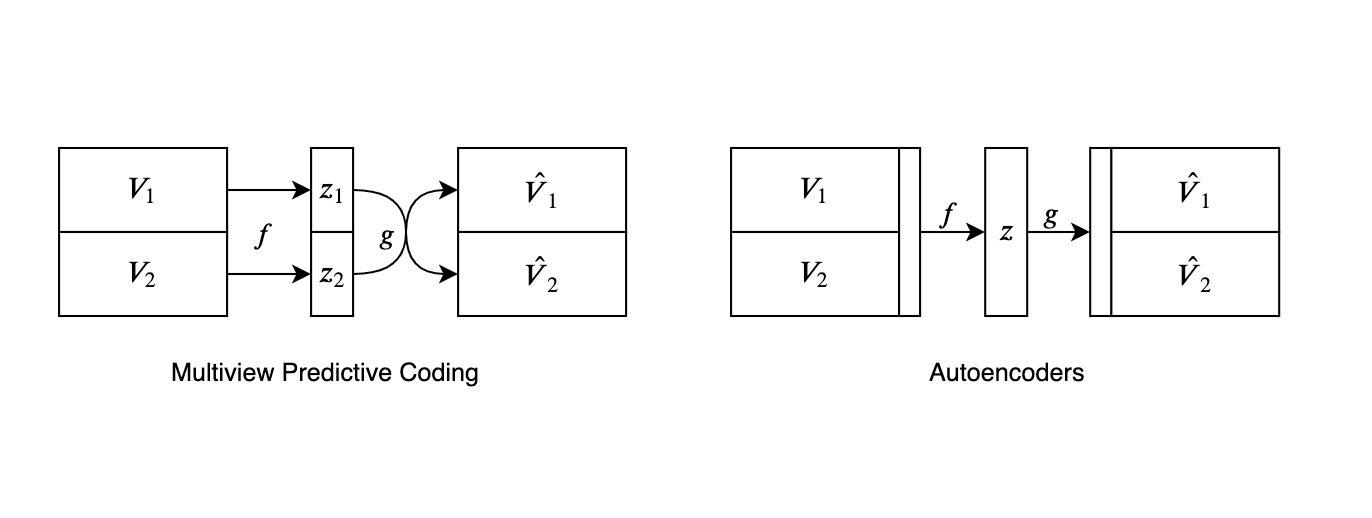The good bits are the ones that are shared between multiple views of the world.

## Contrastive Learning

Multiview Contrastive Learning的基本思想是：将同一个样本的不同view为一个正样本对$\left\{v_{1}^{i}, v_{2}^{i}\right\}_{i=1}^{N}$，对于第i个样本我们能够构造不同样本的不同view为一个负样本对$\left\{v_{1}^{i}, v_{2}^{j}\right\}_{j=1}^{K}$。通过训练一个critic function $h_\theta$来区分正负样本对，从而得到representation. 构造contrast loss为以下：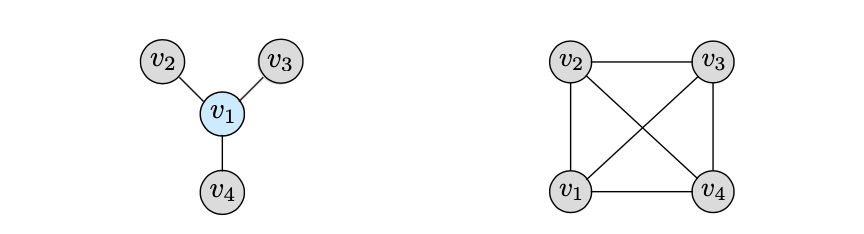# Experiments

• Two established image representation learning benchmarks: ImageNet and STL-10
• Video representation learning tasks with 2 views: image and optical flow modalities
• More than 2 views.

### How does mutual information affect representation quality?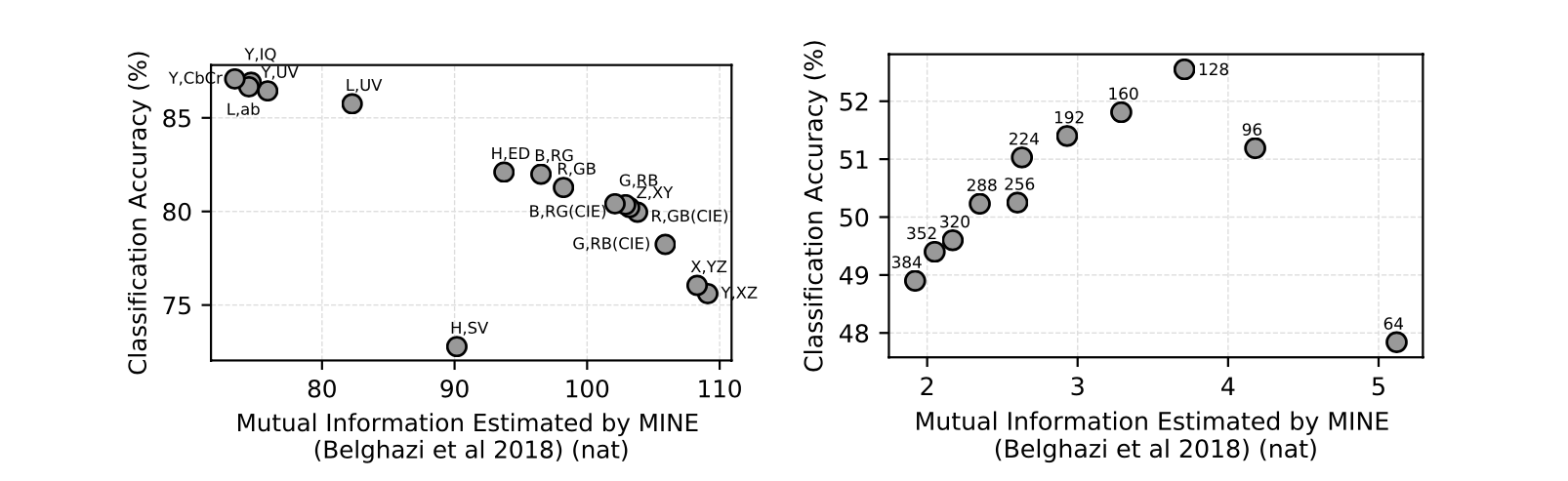Here we see that views with too little or too much MI perform worse; a sweet spot in the middle exists which gives the best representation. That there exists such a sweet spot should be expected. If two views share no information, then, in principle, there is no incentive for CMC to learn anything. If two views share all their information, no nuisances are discarded and we arrive back at something akin to an autoencoder or generative model, that simply tries to represent all the bits in the multiview data.

These experiments demonstrate that the relationship between mutual information and representation quality is meaningful but not direct. Selecting optimal views, which just share relevant signal, may be a fruitful direction for future research.

]]>
<p><strong>Materials</strong></p> <ul> <li><a href="https://arxiv.org/abs/1906.05849" target="_blank" rel="noopener">paper “Contrastive Mult
On MI Maximization for Representation Learning http://www.shihaizhou.com/2020/05/22/On-MI-Maximization-for-Representation-Learning/ 2020-05-22T10:51:40.000Z 2020-06-06T07:44:04.095Z Materials

# Motivation

In this paper we argue, and provide empirical evidence, that the success of these methods cannot be attributed to the properties of MI alone, and that they strongly depend on the inductive bias in both the choice of feature extractor architectures and the parametrization of the employed MI estimators.

## Structure of Introduction

• What is MI: definition
• Fundamental properties of MI
• Firstly, MI is invariant under reparametrization of the variables - namely, if $X^\prime = f_1(X)$ and $Y^\prime = f_2(Y)$ are homeomorphisms (i.e. smooth invertible maps), then $I (X ; Y ) = I (X ^\prime ; Y ^\prime )$.
• Secondly, estimating MI in high-dimensional spaces is a notoriously difficult task, and in practice one often maximizes a tractable lower bound on this quantity.
• Any distribution-free high-confidence lower bound on entropy requires a sample size exponential in the size of the bound.
• In fact, we show that maximizing tighter bounds on MI can result in worse representations.
• In addition, we establish a connection to deep metric learning and argue that this interpretation may be a plausible explanation of the success of the recently introduced methods.

## Multi-view formulation of the MI maximization

In the image classification setting, for a given image $X$, let $X^{(1)}$ and $X^{(2)}$ be different, possibly overlapping views of $X$, for instance the top and bottom halves of the image. These are encoded using encoders $g_1$ and $g_2$ respectively, and the MI between the two representationsg $g_1(X^{(1)})$ and $g_2(X^{(2)})$ is maximized,

where $\hat I$ represents the sample based MI estimator of the true MI $I(X;Y)$ and the function classes $\mathcal{G}_{1}$ and $\mathcal{G}_{2}$ can be used to specify structural constraints on the encoders. One thing to note here is that two encoders $g_1$ and $g_2$ often share parameters.

It gives us plenty of modeling flexibility, as the two views can be chosen to capture completely different aspects and modalities of the data, for example:

• In the basic form of DeepInfoMax $g_1$ extracts global features from the entire image $X^{(1)}$ and $g_2$ local features from image patches $X^{(2)}$, where $g_1$ and $g_2$ correspond to activations in different layers of the same convolutional network. (也就是上一节介绍的global/local-MI Maximization)
• Contrastive multiview coding (CMC) generalizes the objective in to consider multiple views $X^{(i)}$, where each $X^{(i)}$ corresponds to a different image modality (e.g., different color channels, or the image and its segmentation mask).
• Contrastive predictive coding(CPC) incorporates a sequential component of the data. Concretely, one extracts a sequence of patches from an image in some fixed order, maps each patch using an encoder, aggregates the resulting features of the first $t$ patches into a context vector, and maximizes the MI between the context and features extracted from the patch at position $t+k$

# Biases in Approximate MI Maximization

• Encoder: influenced by network structures / parameters.
• Critics: the function tells between the
• Estimators: the lower bound to optimize.

• encoders are bijective. (not reported in this blog)
• encoders that can model both invertible and non-invertible functions. (not reported in this blog)
• different critics: simple / loose bound would lead to high-capacity critics.
• encoder is more important than architectures.

## Setup

Motivation: Our goal is to provide a minimal set of easily reproducible empirical experiments to understand the role of MI estimators, critic and encoder architectures when learning representations via the objective.

Dataset: To this end, we consider a simple setup of learning a representation of the top half of MNIST handwritten digit images. Try to maximize the mutual information of the representation between the representation of the top half and the bottom half of the images. Using bilinear critic: $f(x,y)=x^TWy$.

Evaluation: Following the widely adopted downstream linear evaluation protocol.

## Higher Capacity Critics: Worse Downstream Tasks

In the previous section we have established that MI and downstream performance are only loosely connected. Clearly, maximizing MI is not sufficient to learn good representations and there is a non-trivial interplay between the architectures of the encoder, critic, and the underlying estimators.

Compared 3 different architectures of the critic functions:

• a bilinear critic,
• a separable critic $f(x,y) = \phi_1(x)^⊤\phi_2(y)$ ($\phi_1$, $\phi_2$ are MLPs with a single hidden layer with 100 units and ReLU activations, followed by a linear layer with 100 units; comprising 40k parameters in total)
• an MLP critic with a single hidden layer with 200 units and ReLU activations, applied to the concatenated input [x, y] (40k trainable parameters).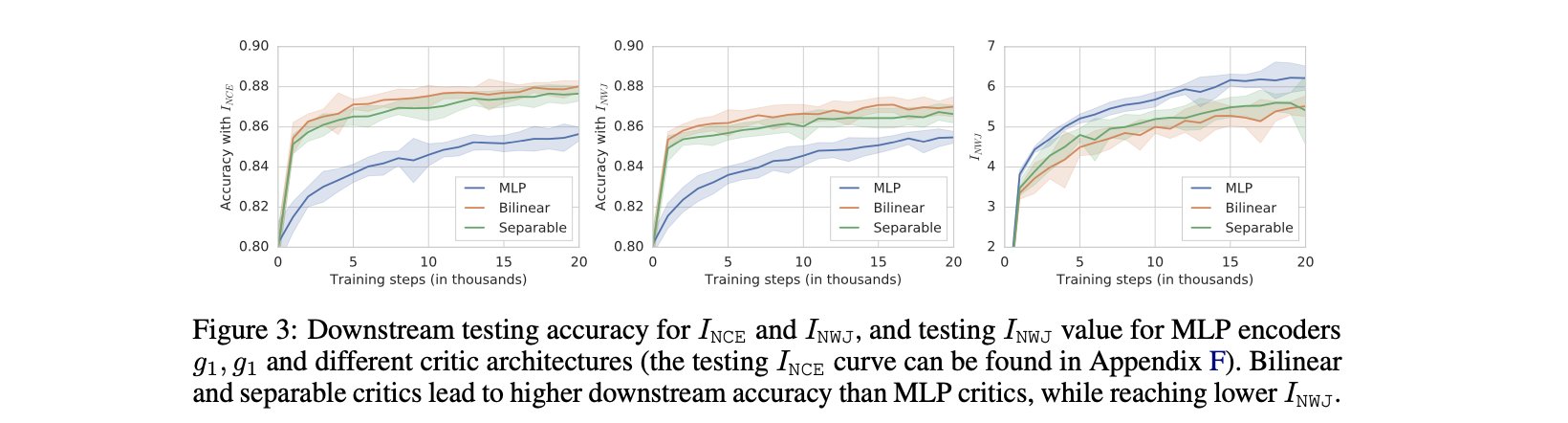## Encoder Architecture: More Important than Specific Estimator

To ensure that both network architectures achieve the same lower bound $I_{EST}$ on the MI, we minimize $L_{t}\left(g_{1}, g_{2}\right)=| I_{\mathrm{EST}}\left(g_{1}\left(X^{(1)}\right) ; g_{1}\left(X^{(2)}\right)\right)-t |$ instead of solving original information maximization problem, for two different values $t = 2, 4$.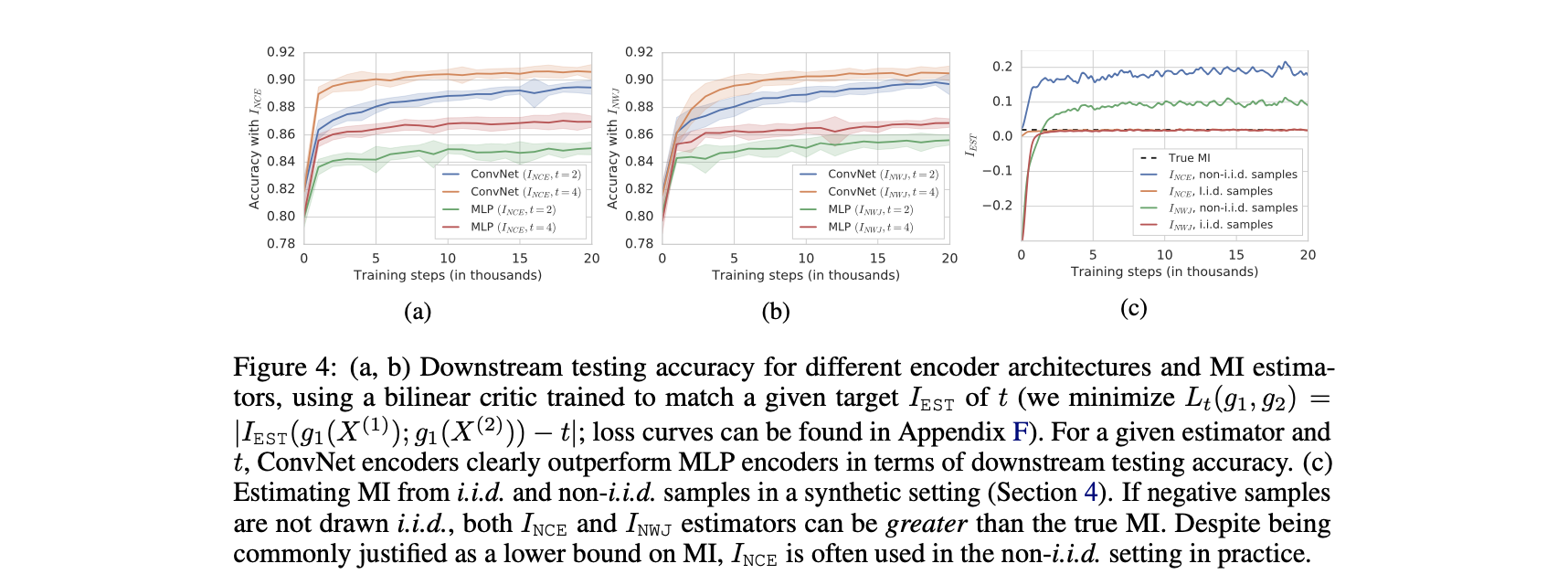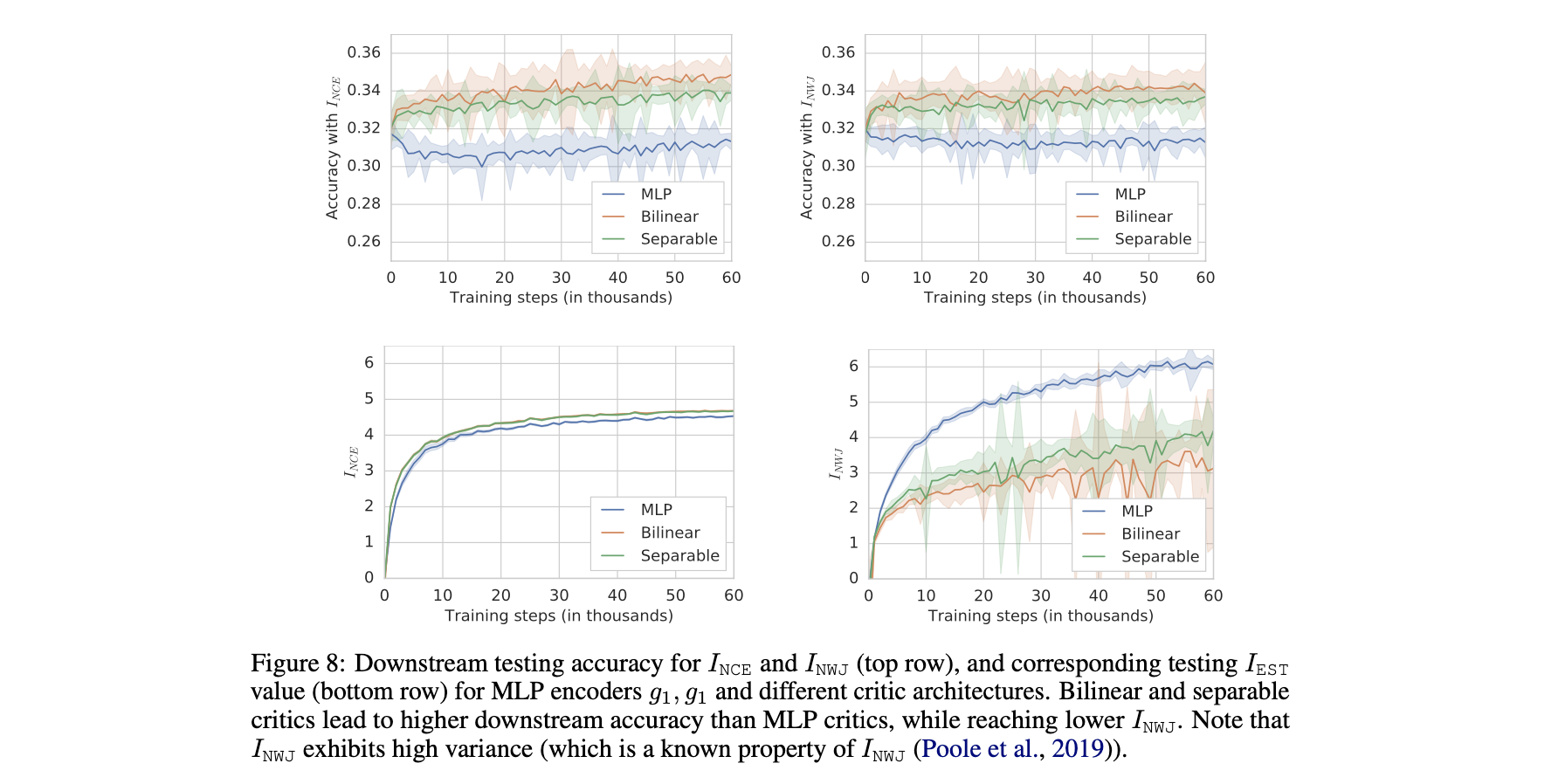# Deep Metric Learning

Given sets of triplets, namely an anchor point $x$, a positive instance $y$, and a negative instance $z$, the goal is to learn a representation $g(x)$ such that the distances between $g(x)$ and $g(y)$ is smaller than the distance between $g(x)$ and $g(z)$, for each triplet. 从这个角度也就不难看出为什么会称MI maximization中的函数为critic function. 而InfoNCE又可以被写作：

# Future Work

### Alternative measures of information

While MI has appealing theoretical properties, it is clearly not sufficient for this task—it is hard to estimate, invariant to bijections and can result in suboptimal representations which do not correlate with downstream performance. Therefore, a new notion of information should account for both the amount of information stored in a representation and the geometry of the induced space necessary for good performance on downstream tasks. One possible avenue is to consider extensions to MI which explicitly account for the modeling power and computational constraints of the observer, such as the recently introduced F-information.

### Going beyond the widely used linear evaluation protocol

While it was shown that learning good representations under the linear evaluation protocol can lead to reduced sample complexity for downstream tasks (Arora et al., 2019), some recent works (Bachman et al., 2019; Tian et al., 2019) report marginal improvements in terms of the downstream performance under a non-linear regime. Related to the previous point, it would hence be interesting to further explore the implications of the evaluation protocol, in particular its importance in the context of other design choices. We stress that a highly-nonlinear evaluation framework may result in better downstream performance, but it defeats the purpose of learning efficiently transferable data representations.

]]>
<p><strong>Materials</strong></p> <ul> <li><a href="https://arxiv.org/abs/1907.13625" target="_blank" rel="noopener">ICLR-2020 paper “On Mut
Variational Auto-Encoders http://www.shihaizhou.com/2020/05/22/Variational-Auto-Encoders/ 2020-05-21T16:06:42.000Z 2020-06-07T08:21:11.194Z Materials

# Latent Variable Models

## Setting

Formally, say we have a vector of latent variables $z​$ in a high-dimensional space $\mathcal Z​$ which we can easily sample according to some probability density function (PDF) $P(z)​$ defined over $\mathcal Z​$. Then, say we have a family of deterministic functions $f (z; \theta)​$, parameterized by a vector $\theta​$ in some space $\Theta​$, where $f:\mathcal{Z} \times \Theta \rightarrow \mathcal{X}​$.

## Target

We wish to optimize $\theta$ such that we can sample $z$ from $P(z)$ and, with high probability, $f (z; θ)$ will be like the $X$’s in our dataset. To make this notion precise mathematically, we are aiming maximize the probability of each $X$ in the training set under the entire generative process, according to:

In VAEs, the choice of this output distribution is often Gaussian, i.e.,

# Variational Autoencoders

VAE的数学推导和Autoencoders并没有太大的关系，为什么会被叫做VAE的原因在于最后从setup推导出的training objective由encoder和decoder两个部分组成，从而成为了AE的形式。

VAE需要解决以下两个问题：（1）如何定义latent variable $z$；（2）如何处理在 $z$ 上的积分.

### How to define the latent variable

• Avoid deciding by hand what information each dimension of $z$ encodes
• Avoid explicitly describing the dependencies—i.e., the latent structure—between the dimensions of $z$.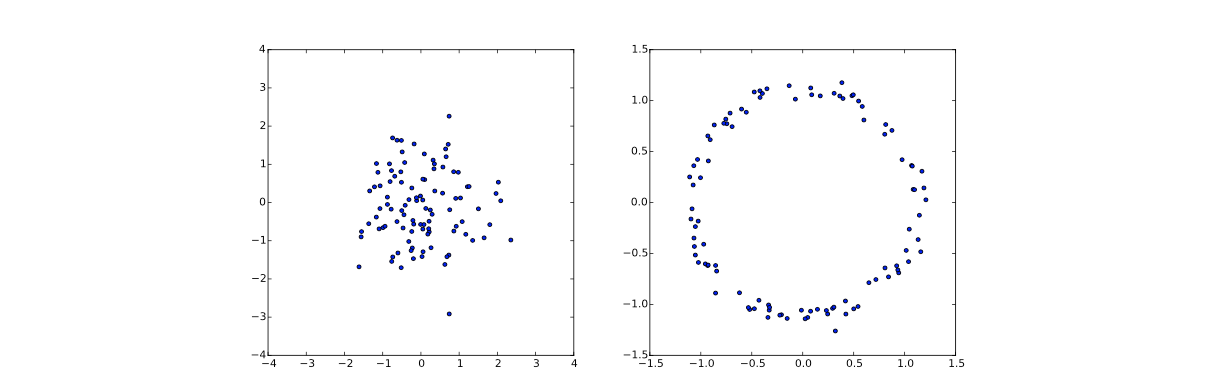## Setting up the Objective

The key idea behind the variational autoencoder is to attempt to sample values of $z$ that are likely to have produced $X$, and compute $P(X)$ just from those. This means that we need a new function $Q(z|X)$ which can take a value of $X$ and give us a distribution over $z$ values that are likely to produce $X$. 这样我们在分布 $Q$ 的帮助下，就非常容易计算$E_{z \sim Q} P(X | z)$，但这只是隐变量$z$在分布$Q$下$P(X)$的估计，和真实的$P(X)$之间是有差距的，为了达到最终目的”optimize $P(X)$”，我们需要”relate $E_{z∼Q}P(X|z)$ and $P(X)$”.

This equation serves is the core of the variational autoencoder, and it’s worth spending some time thinking about what it says . In two sentences, the left hand side has the quantity we want to maximize: $log P(X)$ (plus an error term, which makes $Q$ produce $z$’s that can reproduce a given $X$; this term will become small if $Q$ is high-capacity). The right hand side is something we can optimize via stochastic gradient descent given the right choice of $Q$ (although it may not be obvious yet how). Note that the framework—in particular, the right hand side of the Equation—has suddenly taken a form which looks like an autoencoder, since $Q$ is “encoding” $X$ into $z$, and $P$ is “decoding” it to reconstruct $X$. We’ll explore this connection in more detail later.

## Optimizing the Objective

There is, however, a significant problem with this equation. $E_{z∼Q} [\log P(X|z)]$ depends not just on the parameters of $P$, but also on the parameters of $Q$. However, in the equation above, this dependency has disappeared! In order to make VAEs work, it’s essential to drive $Q$ to produce codes for $X$ that $P$ can reliably decode.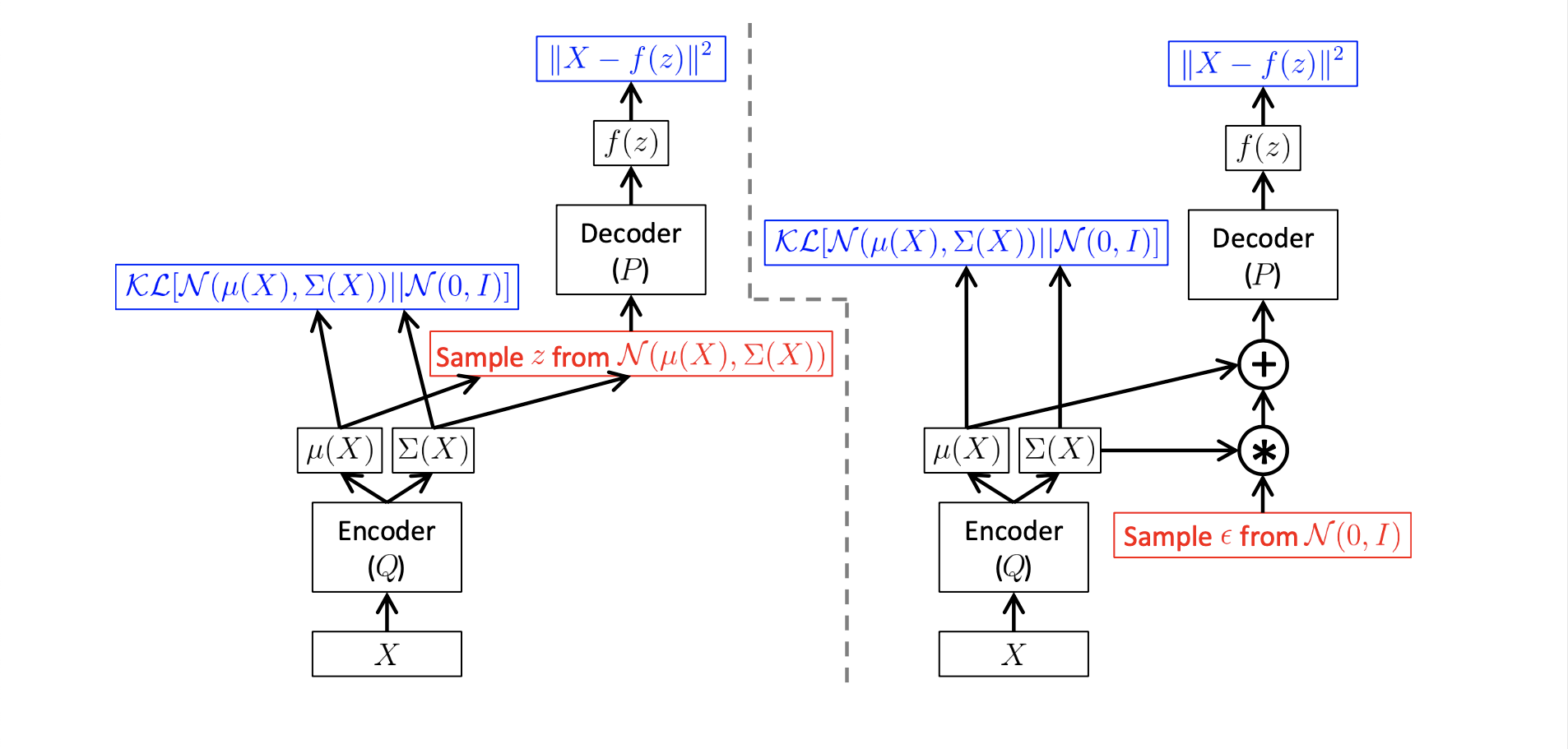]]>
<p><strong>Materials</strong></p> <ul> <li><a href="https://www.youtube.com/watch?v=uaaqyVS9-rM" target="_blank" rel="noopener">Lecture on y